1. Worksheets>
2. Math>
3. Geometry>
4. Surface Area

# Surface Area Worksheets

This page contains a lot of surface area worksheets under various topics. It broadly classified as surface area of prisms, cylinders, pyramids and spheres. Though the cone is a pyramid, we placed it under separate segment for easy identification. Most of the worksheets contain easy, moderate and difficult levels except for few topics. Student must know the formula or technique to solve the problems before taking these surface area worksheets.

Select the Measurement Units

## Surface Area of Prisms

Counting Squares: Rectangular Prism

Each face of a cube has the area 1 cm2. Count the number of faces in each rectangular prism and find the surface area.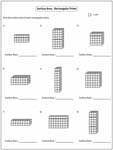Easy:

Moderate:

Surface Area of a Cube Worksheets

Explore these surface area of a cube worksheets to practice computing the total area occupied by the cubes with edge length offered as integers, decimals and fractions. Included here are worksheets to find the edge length and more.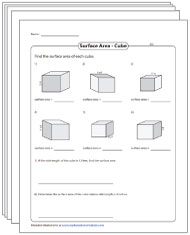Surface Area of a Cube Worksheets

Surface Area of Rectangular Prisms

Find the surface area of each rectangular prism. There are 10 questions in each worksheet. Word problem included.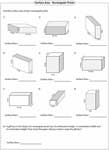Easy:

Moderate:

Difficult:

Challenging:

Surface Area of L-Blocks

Each worksheet has 9 problems finding surface area of L-blocks. You must be careful in finding the dimensions before calculating the surface area.Easy:

Moderate:

Difficult:

Surface Area of a Cylinder

Find the lateral surface area of a cylinder by multiplying the perimeter of a circle with height of the cylinder. To find surface area, add the lateral surface with twice the times of base area.Easy:

Moderate:

Difficult:

Surface Area of Prisms and Cylinders: Level 1

Level 1 prism worksheets contain bases in squares, rectangles and triangles. Cylinders are also included.Easy:

Moderate:

Difficult:

Surface Area of Prisms and Cylinders: Level 2

There are two more bases (trapezoid and parallelogram) along with level 1. These worksheets give you complete practice in finding surface area of prisms.Easy:

Moderate:

Difficult:

Surface Area of Polygonal Prisms

There are 9 regular polygonal prisms in each worksheet. Find the lateral surface area and sum up the result with base areas.## Cone, Sphere and Hemisphere

Surface Area of a Cone

Each worksheet has 9 problems finding surface area of a cone.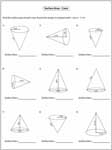Easy:

Moderate:

Surface Area of Sphere and Hemisphere

Each worksheet is a mix of both spheres and hemispheres. There are nine questions in each handout.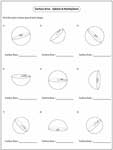Easy:

Moderate:

Difficult:

## Surface Area of Pyramids

Surface Area of Square Pyramids

Finding surface area of square pyramids are quite simple comparing with rest of the pyramids. Each worksheet has 6 problems with a solved example.Easy:

Moderate:

Difficult:

Surface Area of Polygonal Pyramids

Surface Area = Base Area + (0.5 * Perimeter * Slant Height). This is the most general formula to find the surface area of regular polygonal pyramids. The bases are square, triangle, pentagon and hexagon.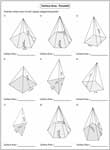## Mixed and Compound Shapes

Surface Area of Mixed Shapes

The mixed shape worksheets contain prisms, cylinders, cones and spheres. You must be aware of all formulas related to the shapes.Easy:

Moderate:

Difficult:

Surface Area of Combined Shapes

Finding surface area of compound shapes are quite easy, but you should remember to delete the overlapping area.